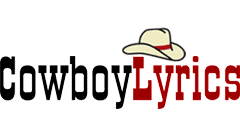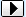MENU Request Lyrics   Add/Correct Lyrics   Links Page   Contact Us   Privacy Policy   DMCA Policy MERCHANDISE Country CDs   Country Sheet MusicBROWSE LYRICS AND TABS BY ARTIST/BAND NAME:SEARCH:A B C D E F G H I J K L M N O P Q R S T U V W X Y Z ## WHERE WE ARE lyrics - The LumineersWhere we are (Where we are)
I don't know where we are (Where we are)
But it will be okay
Where we are (Where we are)
I don't know where we are (Where we are)
But it will be okay

Layin' on the table like I wasn't even there
Holdin' on the steering wheel and coming up for air
What was I
Drivin' in the rain
What was I

Starin' at the ceiling fan and feelin' far away
Leanin' on to reasons like it wasn't even fair
What was I
Drivin' in the rain
What was I

Where we are
I don't know where we are
But it will be okay
Where we are
I don't know where we are
But it will be okay

Crashed the car in Arizona on the interstate
People pullin' over cryin' thinkin' we were dead
What was I
Drivin' in the rain
What was I

I know who you wanted me to be

Where we are
I don't know where we are
But it will be okay
Where we are (Where we are)
I don't know where we are (Where we are)
But it will be okay

Where we are (Where we are)
I don't know where we are (Where we are)
But it will be okay
Where we are (Where we are)
I don't know where we are (Where we are)
But it will be okay

Where we are (Where we are)
I don't know where we are (Where we are)
But it will be okay
Where we are (Where we are)
I don't know where we are (Where we are)
But it will be okay

Where we are
I don't know where we are
But it will be okay

The Lumineers Chords

The Lumineers Chords